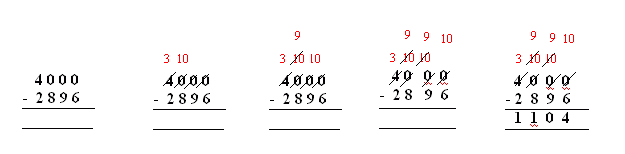Email us to get an instant 20% discount on highly effective K-12 Math & English kwizNET Programs!

#### Online Quiz (WorksheetABCD)

Questions Per Quiz = 2 4 6 8 10

### Grade 3 - Mathematics6.3 Subtraction Across Zeroes (WIZ Math)

 Sometimes when you need to regroup, there is a zero in the next place. Then you need to regroup or rearrange the numbers in a different way. Method: Line up the numbers in their place value columns. Subtract the digits in the ones place. Since you cannot take away from zero, you need to regroup. That is borrow from tens place. If the tens digit is also zero then borrow from hundreds digit. If the hundreds digit is also zero then borrow from thousands digit and so on... After regrouping or rearranging subtract each column starting with the ones, tens, hundreds and so on.... Example:Regroup the thousands digit: 4 thousands as 3 thousands and 10 hundreds. Regroup the hundreds digit: 10 hundreds as 9 hundreds and 10 tens. Regroup the tens digit: 10 tens as 9 tens and 10 ones. Subtracting each column starting with ones, tens, hundreds and thousands gives 1104 Answer: 1104 Directions: Subtract the following. Show your work with regrouping numbers. Also write at least 5 examples of your own. For more worksheets: 1) Click Here 2) Refresh every time and you will see different worksheet for unlimited practise.
 Question 1: This question is available to subscribers only! Question 2: This question is available to subscribers only! Question 3: This question is available to subscribers only! Question 4: This question is available to subscribers only!

#### Subscription to kwizNET Learning System offers the following benefits:

• Unrestricted access to grade appropriate lessons, quizzes, & printable worksheets
• Instant scoring of online quizzes
• Progress tracking and award certificates to keep your student motivated
• Unlimited practice with auto-generated 'WIZ MATH' quizzes